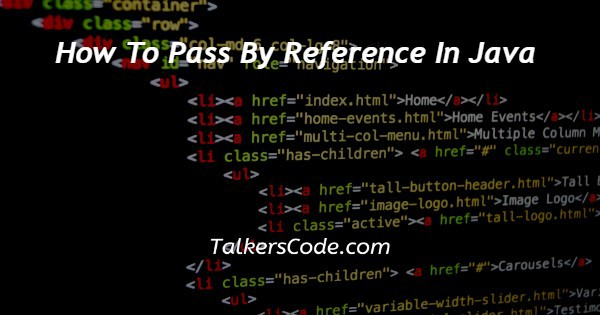# How To Pass By Reference In Java

Last Updated : Sep 7, 2023In this article we will show you the solution of how to pass by reference in java, when passing a parameter to a method in Java, a copy of the value of the parameter is normally supplied as the parameter's value.

However, Java has a method for passing objects by reference that enables method modifications to the original object.

You must be aware that objects actually represent references that are held in memory in order to transmit an object through reference in Java.

An object reference rather than the actual object is supplied when it is passed as a parameter.

This means that any modifications that are made to an object inside the function will also impact the object beyond the method.

The object's reference can be used as a parameter in an approach and its properties or methods can be called on it to accomplish pass-by-reference behavior.

Any modifications made to the object inside the method will then be mirrored in the original object in this manner. Now we'll talk about the Java concept of passing by reference.

## Step By Step Guide On How To Pass By Reference In Java :-

```import java.util.Random;
class MyClass {
int value;
MyClass(int value) {
this.value = value;
}
void generateRandomValue() {
Random random = new Random();
value = random.nextInt(100);
}
void multiplyValue(int factor) {
value *= factor;
}
}
class Main {
public static void main(String[] args) {
MyClass obj = new MyClass(10);
// Pass the reference of the object
modifyObject(obj);
// The changes made inside the method are reflected in the original object
System.out.println("Modified value: " + obj.value); // Output: Modified value: 20
obj.generateRandomValue();
System.out.println("New random value: " + obj.value); // Output: New random value: [some random number]
obj.multiplyValue(3);
System.out.println("Multiplied value: " + obj.value); // Output: Multiplied value: [value multiplied by 3]
}
public static void modifyObject(MyClass obj) {
// Modifying the object's value
obj.value = 20;
}
}```
1. You can see that we have written some Java code that demonstrates passing by reference in Java.
2. We begin with importing the Random class from the java.util package, which enables us to generate random integers within our code.
3. A type-int instance variable is used to define the MyClass class.
4. The constructor for the MyClass class is MyClass(int value), which accepts an integer parameter & sets it to the value of the variable.
6. generateRandomValue(): With the use of this method, we can create a new instance for the Random class, produce an integer at random between 0 and 100, and then assign that number to the value of a variable.
7. Using the multiplyValue(int factor) method, we can increase the value variable by the specified factor, an integer parameter.
8. After that, we have the Main class, which is where our program begins.
9. We construct an object of the MyClass class called obj and initialize it with the number 10 inside the main method.
10. The modifyObject(obj) function is then called, passing the obj object as an argument. By using this method, the object's value is changed to 20.
11. After making changes to the object, we display the modified value from obj.value, which returns a Modified value: 20.
12. The generateRandomValue() function of the obj object is then called. By using this method, a fresh random value is created and assigned to obj.value.
13. We next execute the print command for the new random value of obj.value, which produces the result New random value: [some random integer].
14. Finally, we invoke the obj object's multiplyValue(3) method, which multiplies the value by three.
15. Finally, we show the multiplied value of obj.value, which produces the result Multiplied value: [value multiplied by 3].

## Conclusion :-

As a result, we have successfully learned the Java idea of passing by reference.

We also discovered that we could change the value property of an object, which was mirrored in the original object, by making an instance of the MyClass class & sending it as an argument to the modifyObject method.

We also expanded the code to demonstrate other features, such as creating random values & multiplying the value of the object.

I hope this article on how to pass by reference in java helps you and the steps and method mentioned above are easy to follow and implement.